Certificate

### Learn on Scaler Topics and get certified.# Non Linear Data Structures

Learn about Non Linear Data StructureChallenge Inside! : Find out where you stand! Try quiz, solve problems & win rewards!

## Abstract

A Non-Linear Data Structure is one in which it's elements are not connected in a linear fashion, as suggested by it's name itself. In such a data structure elements might be connected in a hierarchy manner like a tree or graph, or it may be non hierarchical like in a LinkedList. Non-linear data structures have more complex implementation, than it's linear counterparts, but fret not, as we discover more about this topic together!

## Scope

• This article introduces Non-Linear Data structure, explores the examples of non linear data structure, and goes through the differences between linear and non linear data structures.
• This article does not cover the implementations of the mentioned non linear data structures.

# Takeaways

The main advantage of non-linear data structure is that it uses memory very efficiently than linear data structures.

## An intuitive introduction to Non Linear Data Structure

Suppose, John and Sarah are two high school students. Their class teacher assigned them to give a detailed list consisting of the names of the Faculties in their year, along with their departments.

John decides to make a table consisting of name of each person along with his/her department and designations.

Member NameDesignation
JanePrincipal
MarillaHOD, Science Dept
AnneTeacher, Physics
GilbertTeacher, Chemistry
MoodyTeacher, Maths
MathewHOD, Commerce Dept
JerryTeacher, Accountancy
MaryTeacher, Economics

Sarah, on the other hand decides to make a tree diagram which shows all the faculties along with their designations: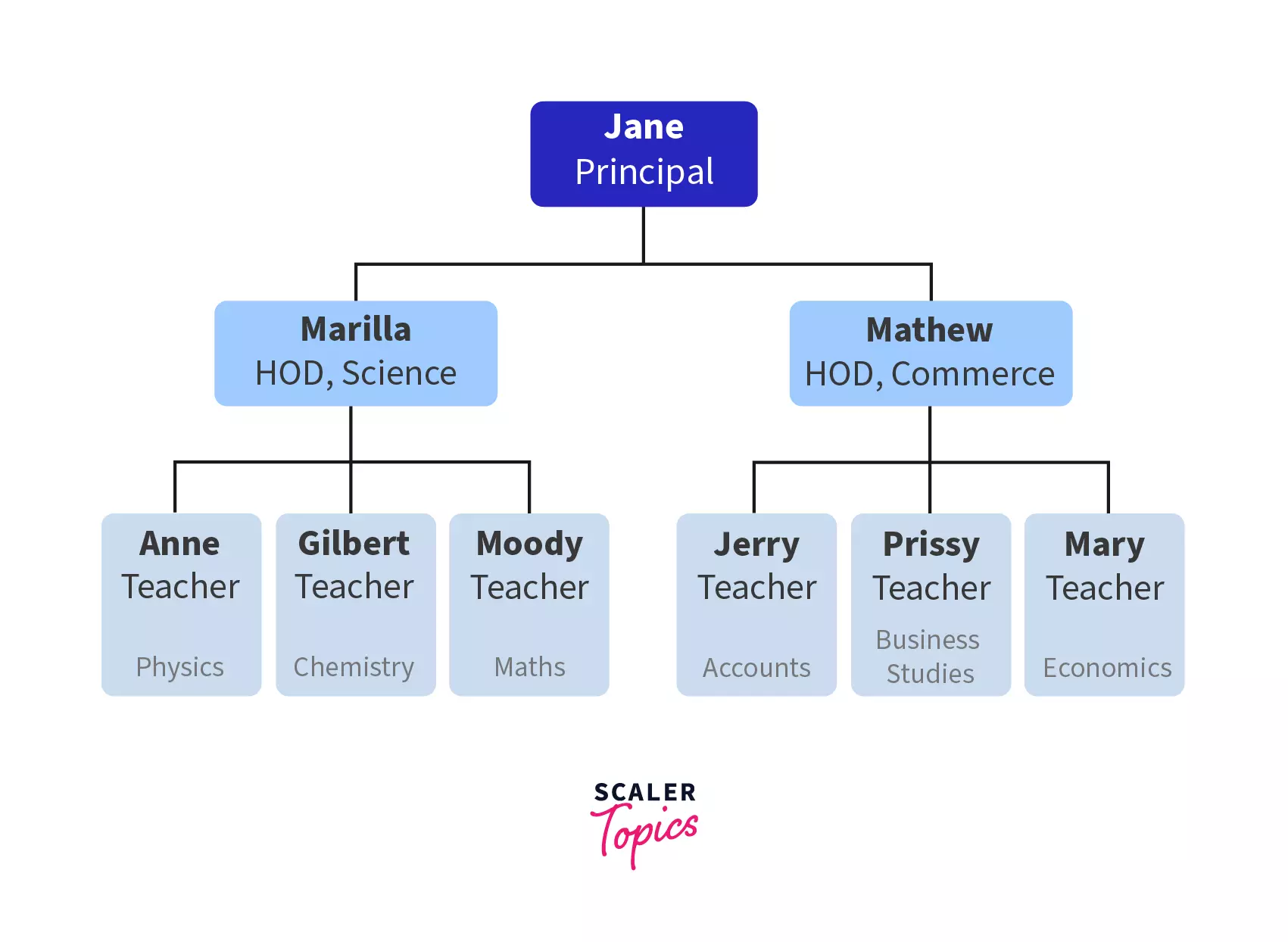### Q. Who do you think will get a better grade in their assessment?

It's Sarah, because she has represented the relationship between the faculties while John has only provided a one-sided list that does not show who works under whom. John's list is a linear data structure as you might have guessed, while Sarah's tree is a non linear data structure.

### Let us now analyse the key points of a Non-Linear Data Structure:

• Elements are not arranged sequentially.
• One element can be connected to multiple elements.
• There might be a hierarchical structure present.
• Here, memory is not allocated in a contiguous manner unlike linear data structure.

## Examples of Non Linear Data Structure

Some examples of non-linear data structures are LinkedLists, Trees and Graphs. We'll now go through each of them and understand why they are called non linear data structure.

A LinkedList is a collection of nodes. Each node contains a data field, and the address (reference) to the next node.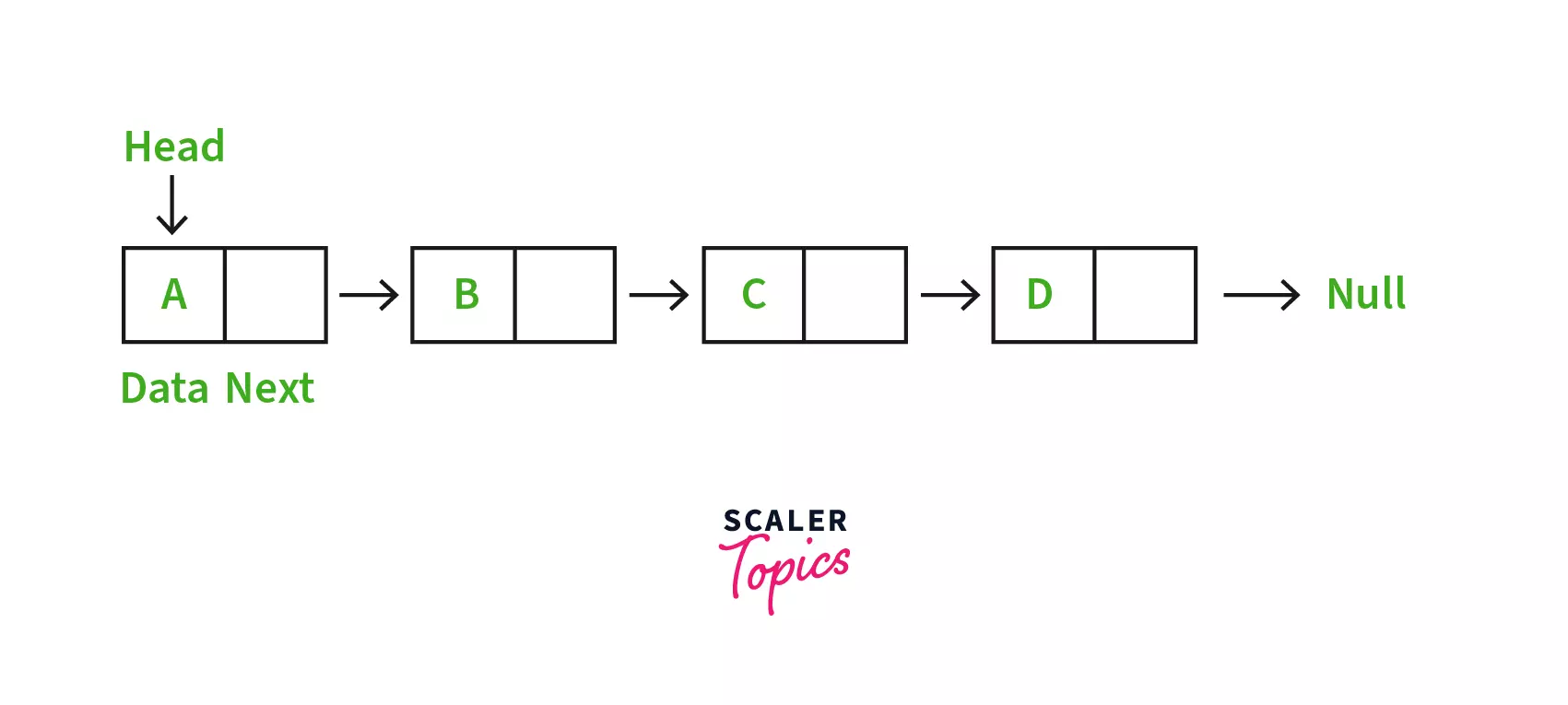### Q. Why is LinkedList Non Linear?

Even though, it might seem that LinkedList should be Linear due to it's sequential connection of elements, you must remember that there is no contiguous memory structure in a LinkedList. All the elements of a LinkedList are spread across the memory in a Non Linear fashion, hence it is a Non Linear Data Structure.

## Tree

As you might have figured it, tree is a data structure that is both non linear as well as hierarchical. Here elements are arranged in multiple levels, and each level can be completely or partially filled. Let us now go through some of the basic terminologies of a tree-

• Root - The topmost node of the tree
• Parent - Each node is a parent of the nodes connected to it, below.
• Child - Each node that is a descendant of another node is called child of that node.
• Siblings - Nodes with same parent
• Leaf - The nodes in the last/bottom-most level of the tree
• Edge - Link connecting two nodes.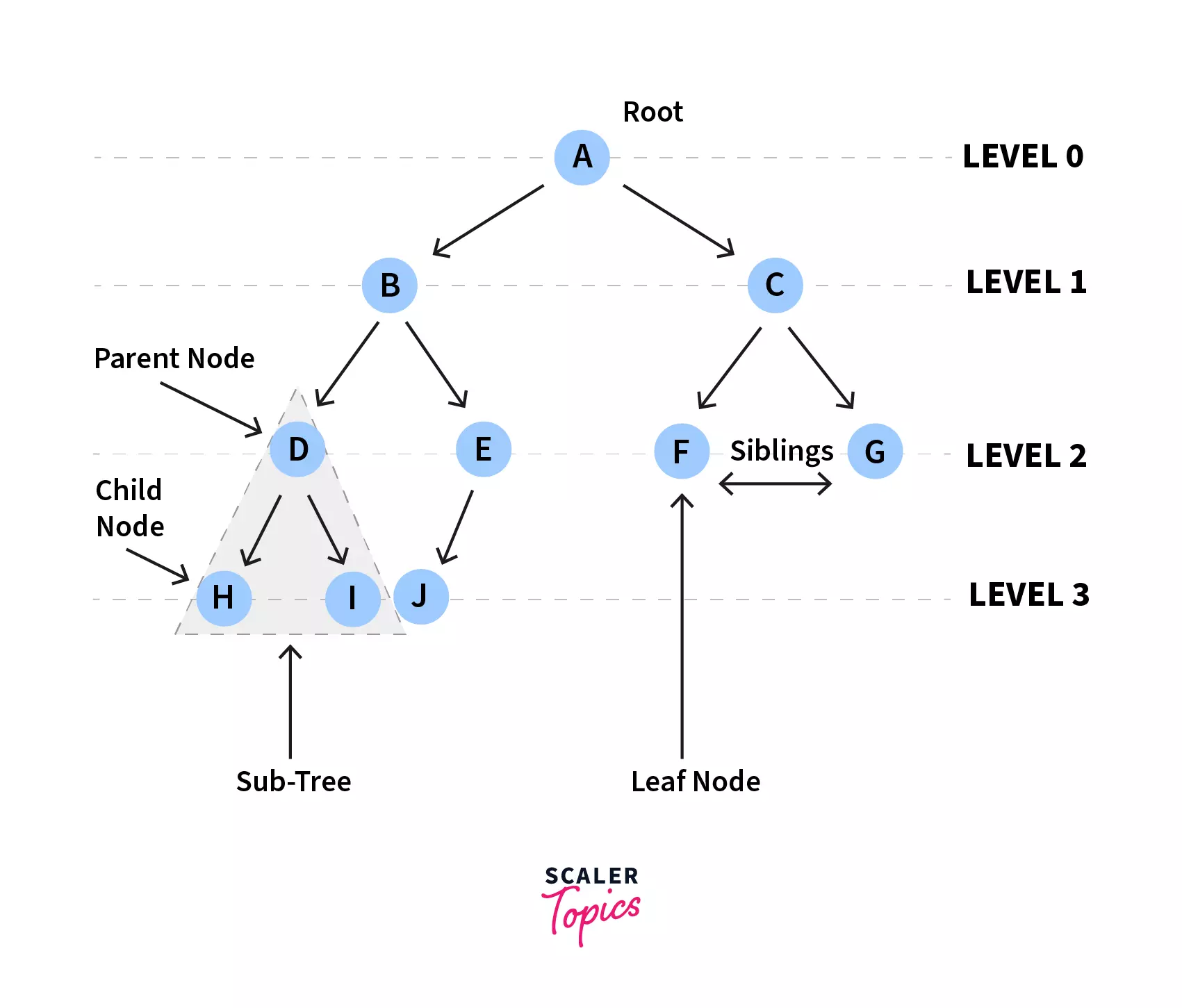• ### General Tree

A tree that can contain any number of subtrees is known as a general tree.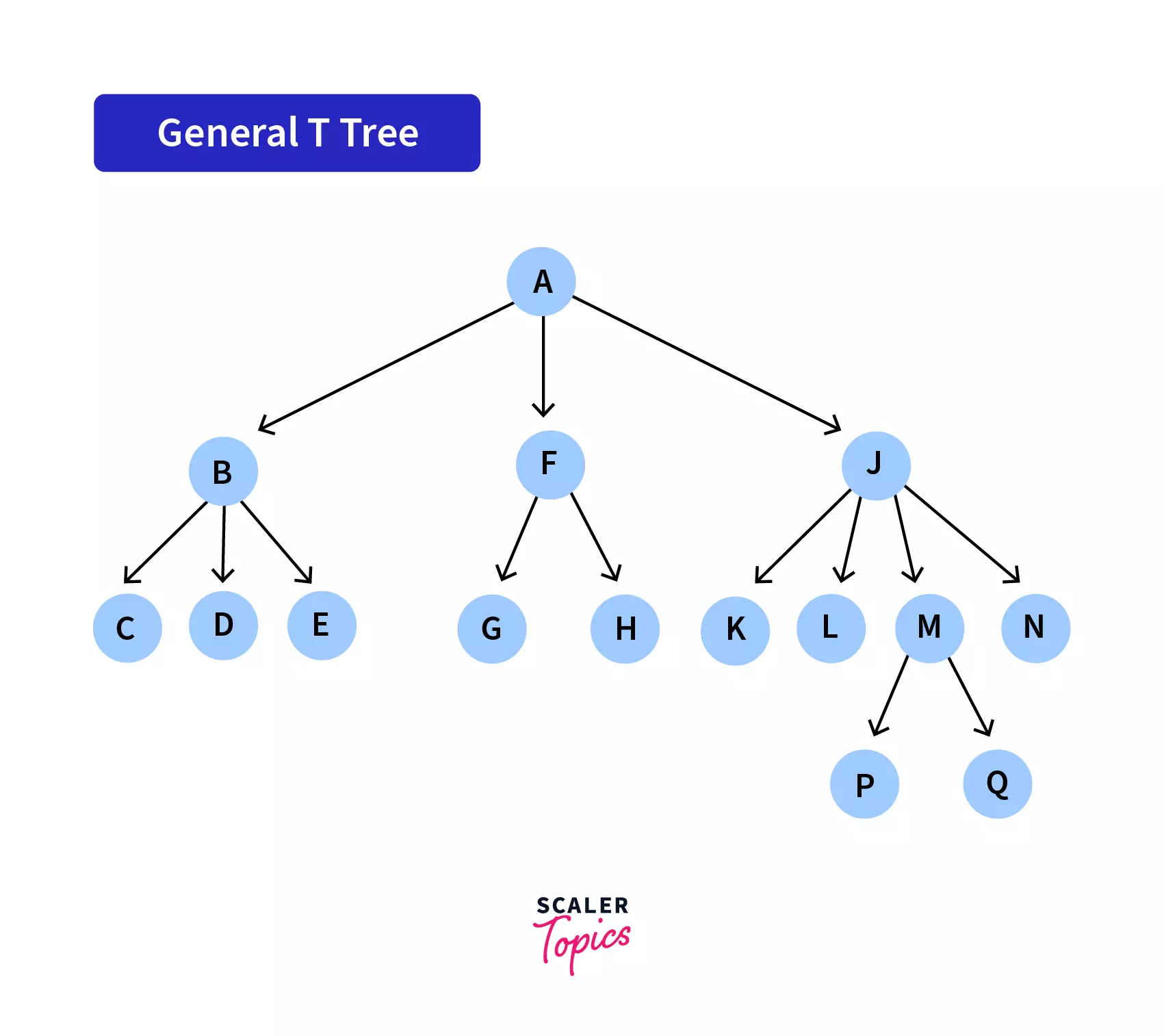• ### Binary Tree

A tree where each node has atmost two children (known as left child and right child) is known as a binary tree. In the figure below, each subtree has either one or two child.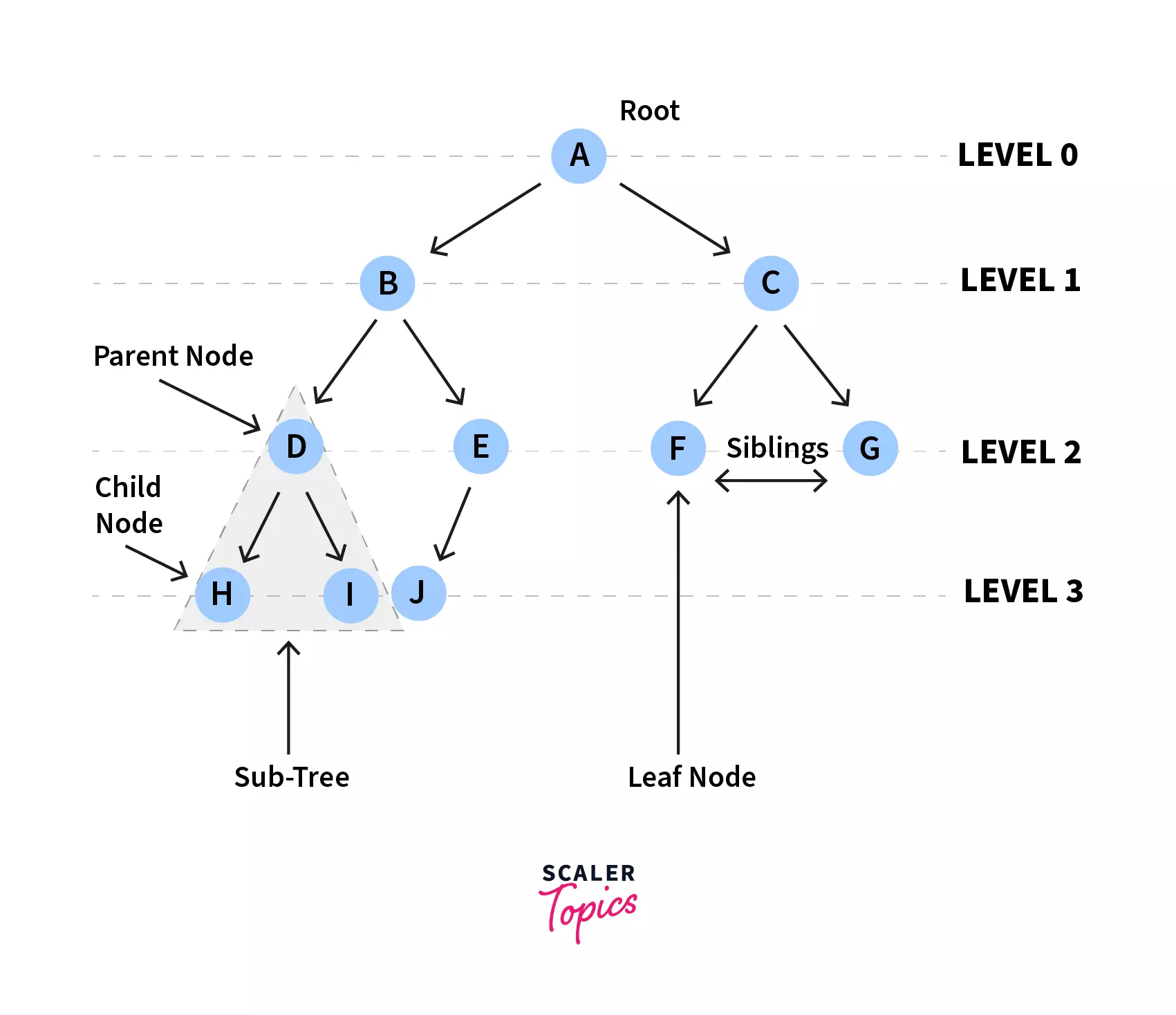• ### Binary Search Tree

Binary Search tree is a special kind of binary tree that holds the following properties:

1. All the nodes in the left subtree holds key with value lesser than the node's key value.
2. All the nodes in the right subtree holds key with value greater than the node's key value.

The first figure is a Binary Search Tree, since it holds the above two properties.

However, for the second figure, 2 lies in the right subtree of 3 and has a lesser value than 3, whereas in a Binary Search Tree, all the nodes in the right subtree should hold key with value greater than the node's key value. Hence, the second figure is not a Binary Search Tree.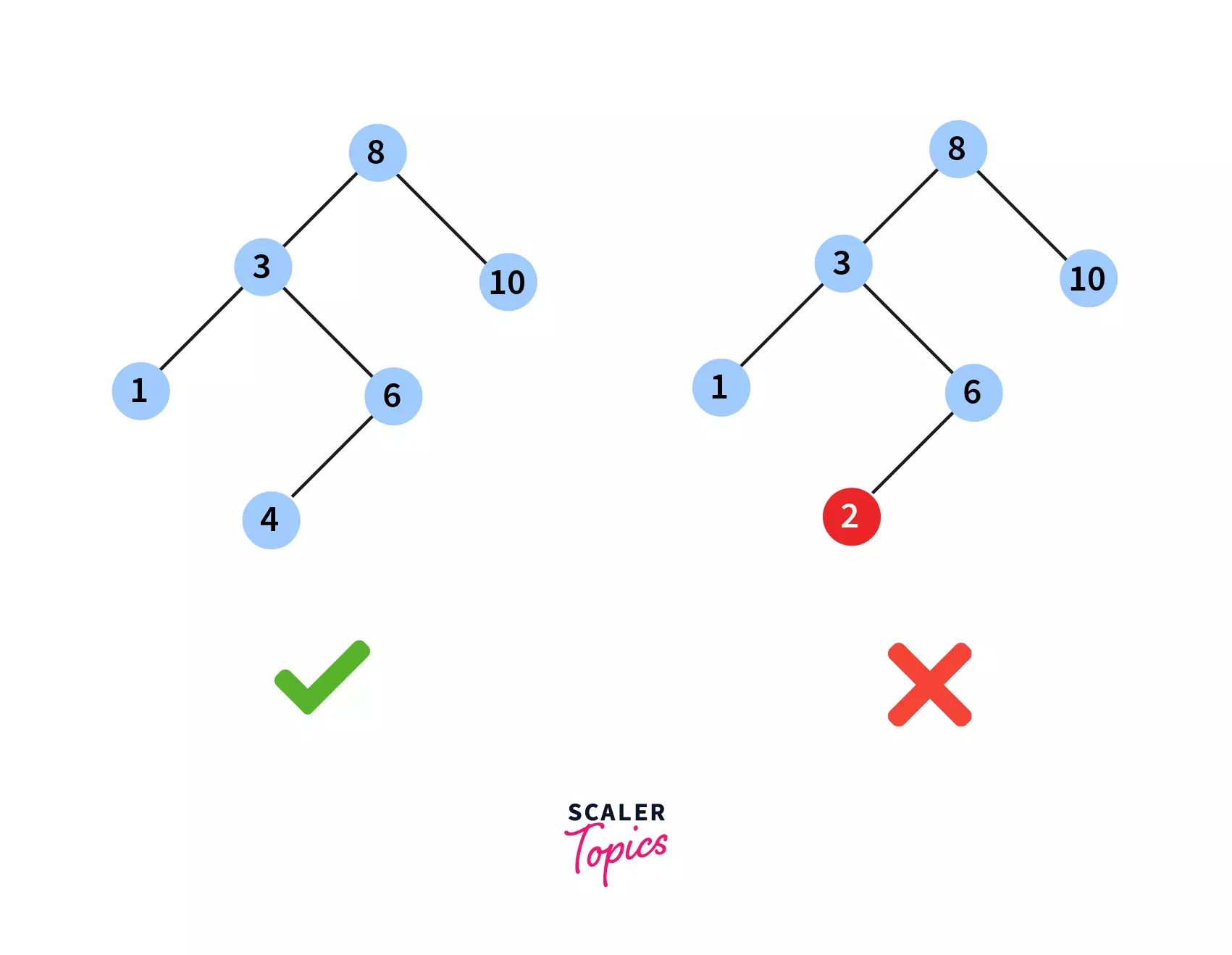• ### AVL Tree

AVL Tree is a special kind of Binary Search Tree where height difference of the left and right subtrees is less than or equal to one. Eg - In the figure given below, let's analyse height difference of the left and right subtrees for all the subtrees: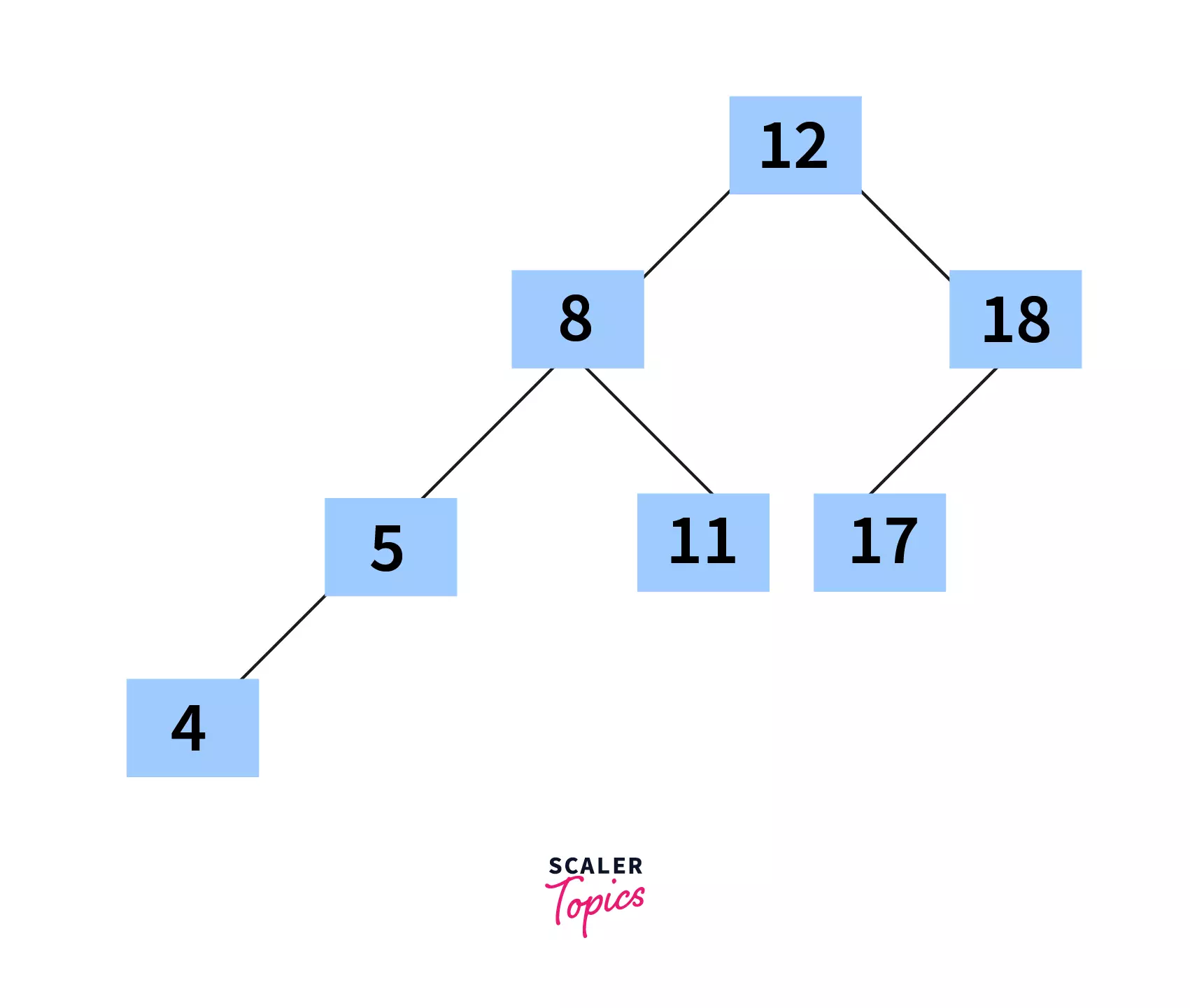Parent Node of SubtreeHeight of left subtreeHeight of right subtreeHeight difference
12321
8211
18101
5101
11000
17000
4000

As, we can see, the maximum height difference between any left and right subtree is one. Thus the below tree is an AVL Tree.

### 2-3 Tree

A tree where every non leaf node has either of the following two properties:

1. One data elements and two children.
2. Two data elements and three children.

Below is a 2-3 tree: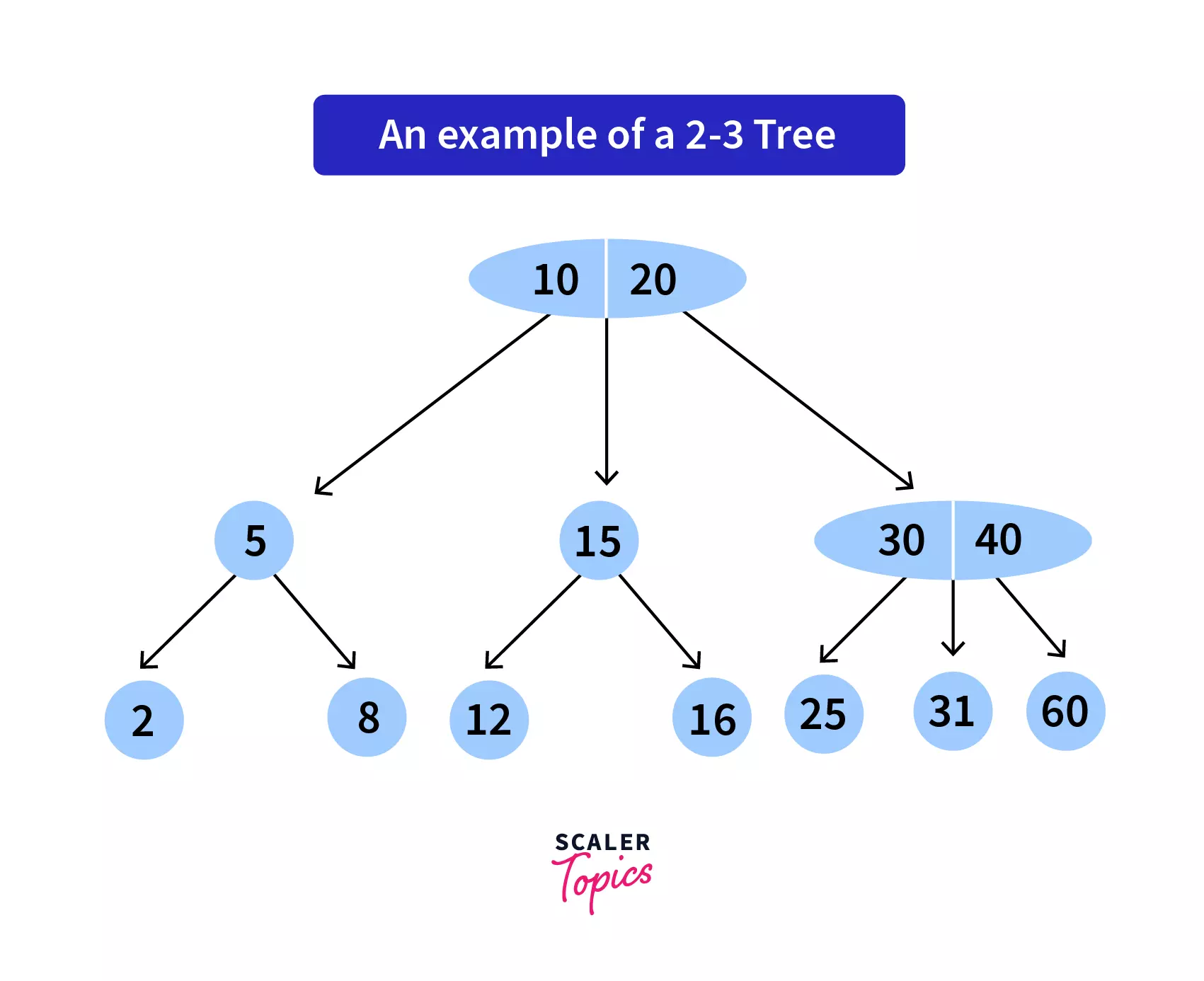• ### B Tree

A B Tree helps to store data in sorted order and is commonly used in database or file systems. Below are some of the properties that a B Tree of order m holds:

1. There can be atmost m children for each node.
2. There should be atleast ⌈m/2⌉ child nodes for each non-leaf node (except root).
3. The root should contain minimum 1 key.
4. Depth of every leaf is same.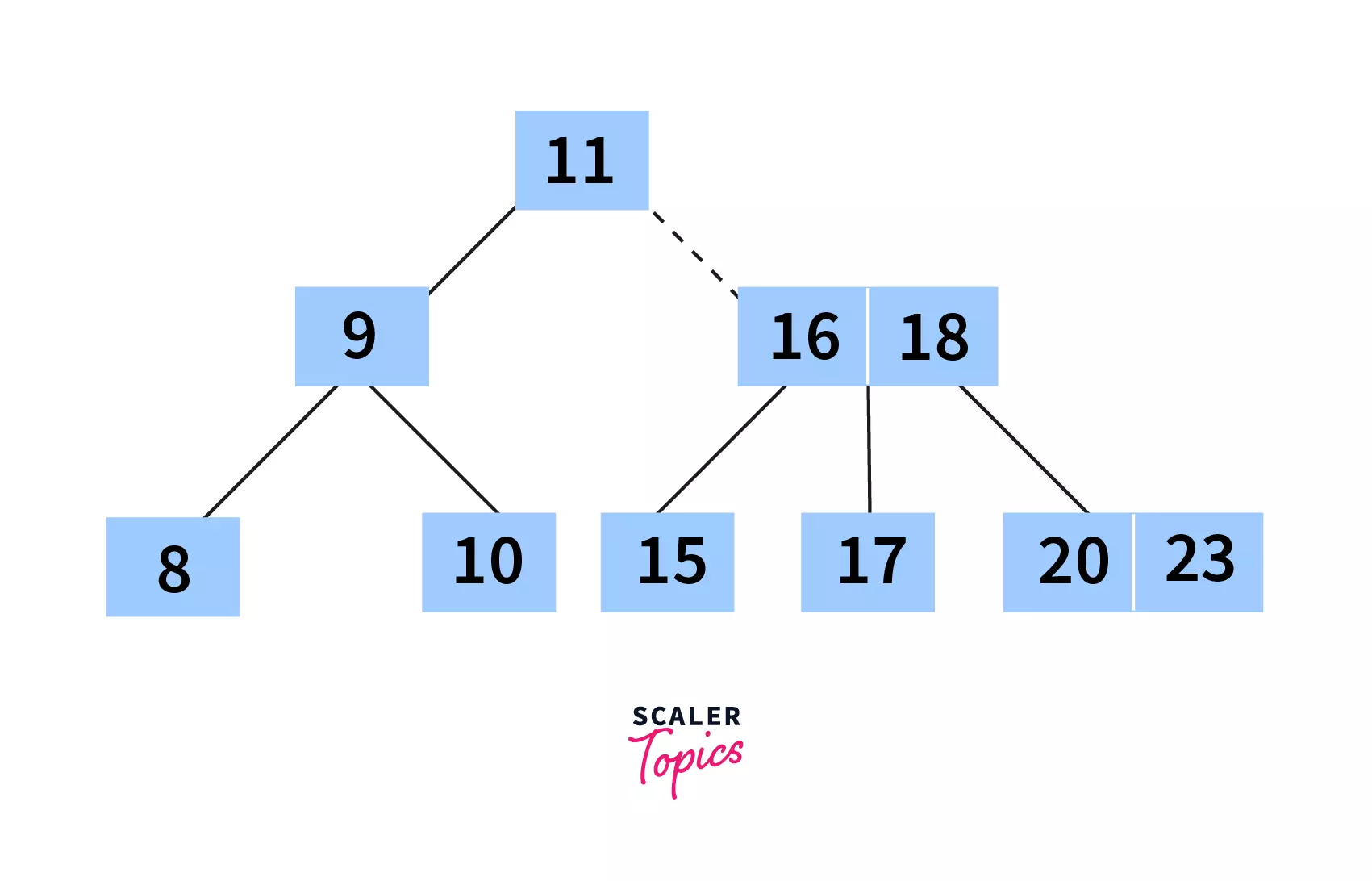### B+ Tree

B+ tree is an extension of B Tree. It often contains large number of children per node.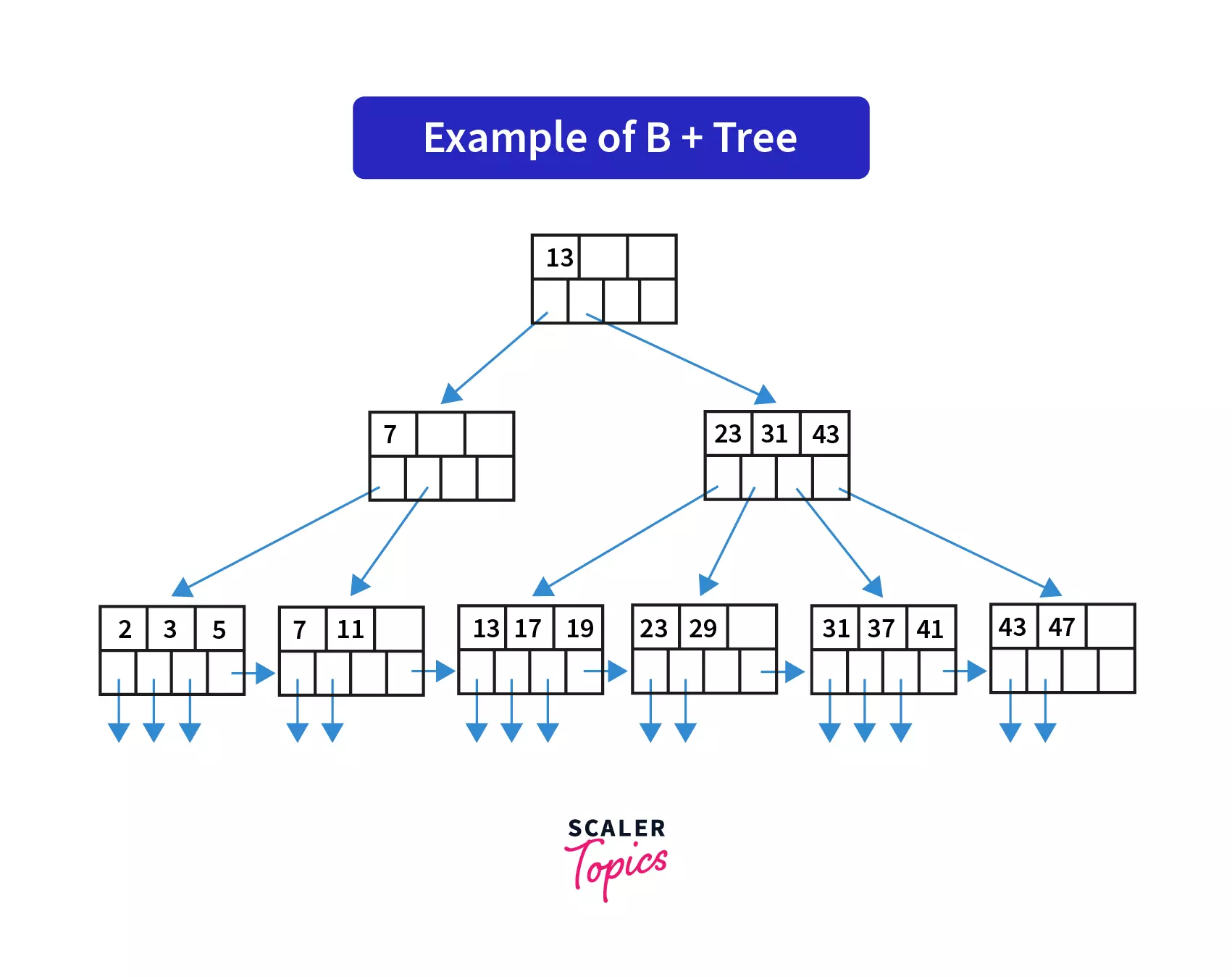## Graph

A graph is a non linear data structure that consists of the following:

• Nodes - It is a finite set consisting of the vertices.
• Edges - A finite set of ordered pair in the form of (x,y) that connects any two vertices of the graph.

• ### Directed Graph :

As the name suggests, this type of graph contains directed edges, i.e it's edges are pointing in a particular direction. The graph can be traversed only in the direction pointed by the edges.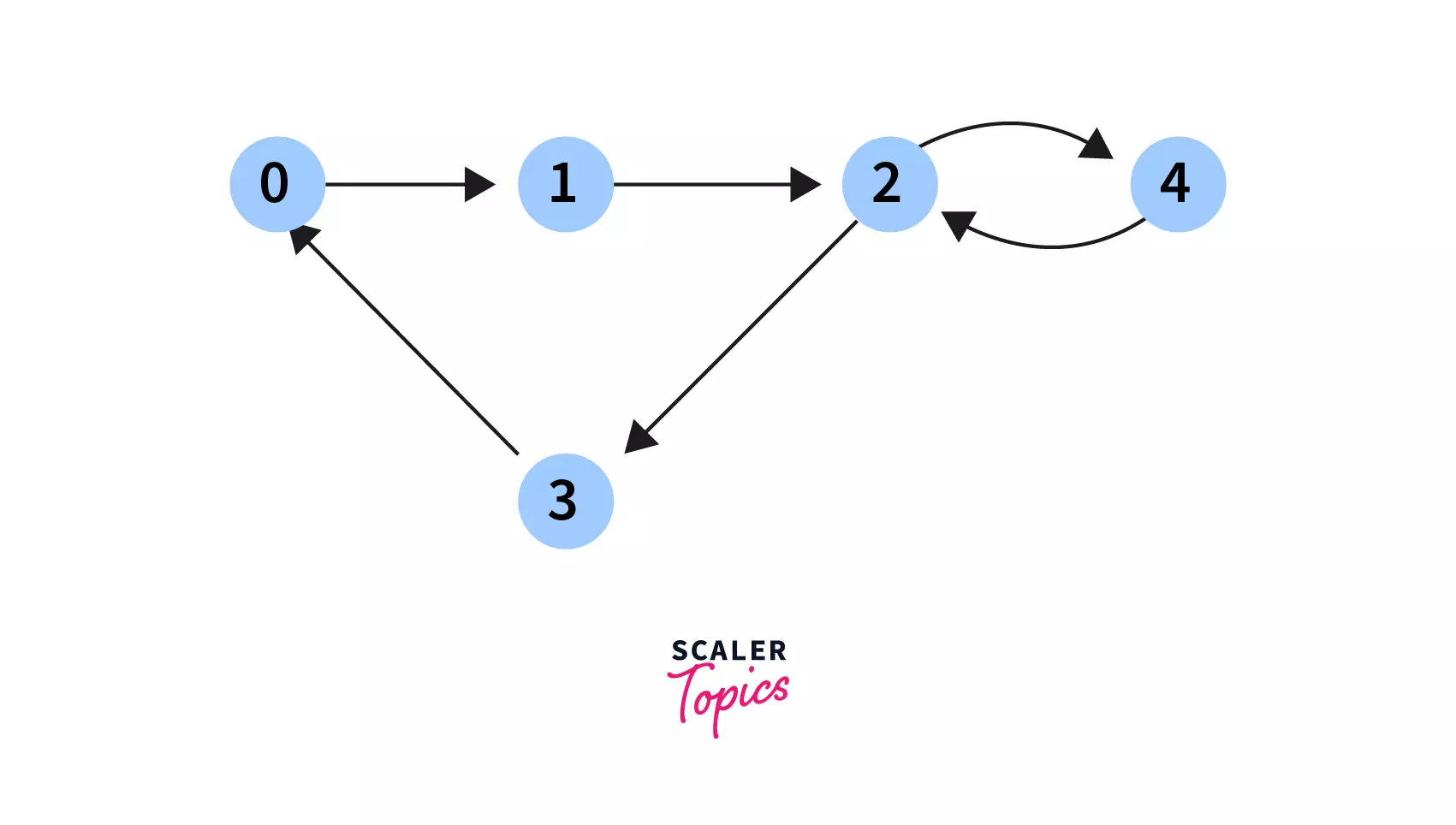### Undirected Graph :

This type of graph does not contain any direction associated with it's nodes, that is it's edges are nondirectional. We can traverse an undirected graph in either direction.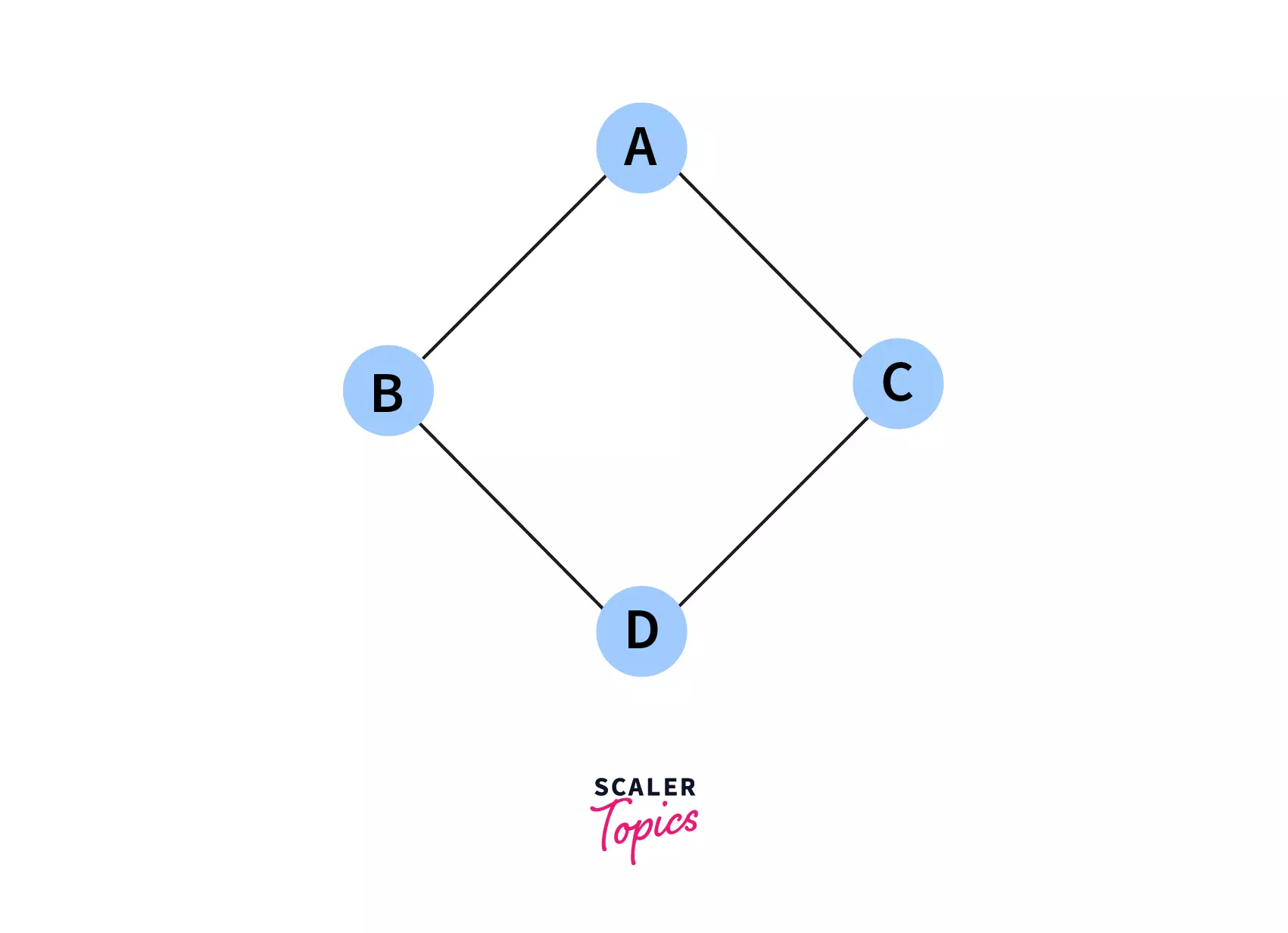### Q. What is the difference between a directed an undirected edge?

A directed adge points towards a particular direction and is represented by an arrow on the edge. An undirected edge does not point towards any direction and is represented by a straight line.

### Weighted Graph :

A graph that has a weight, i.e a certain numeric value associated with it's edges is known as a weighted graph. Weighted graphs can be both directed as well as undirected.

This weighted edges can be used to solve a number of problems, such as minimum cost path to go from one city to another, where the weight of the edges represent the cost to travel between the connected nodes.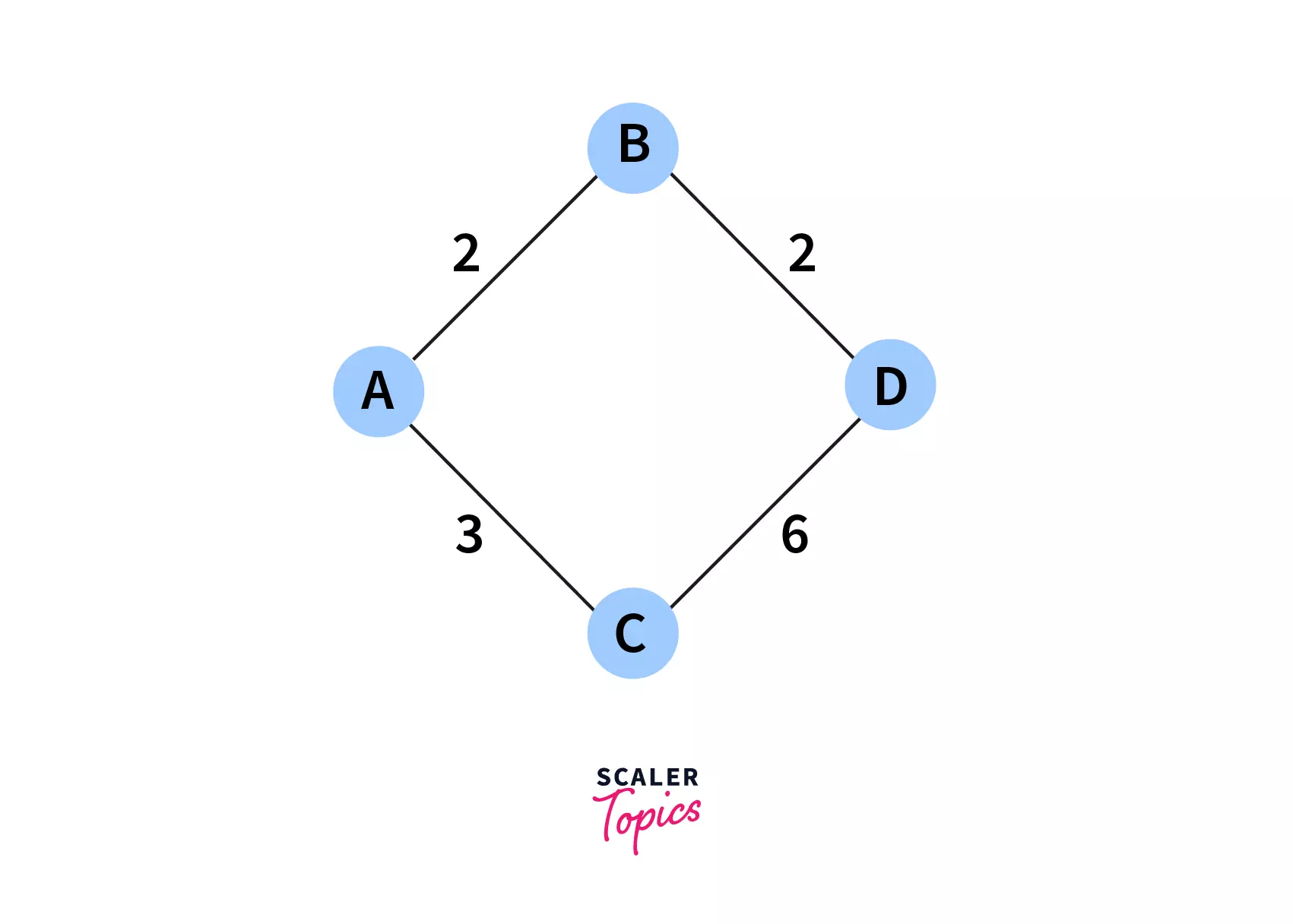## Differences between the Linear data structure and non-linear data structure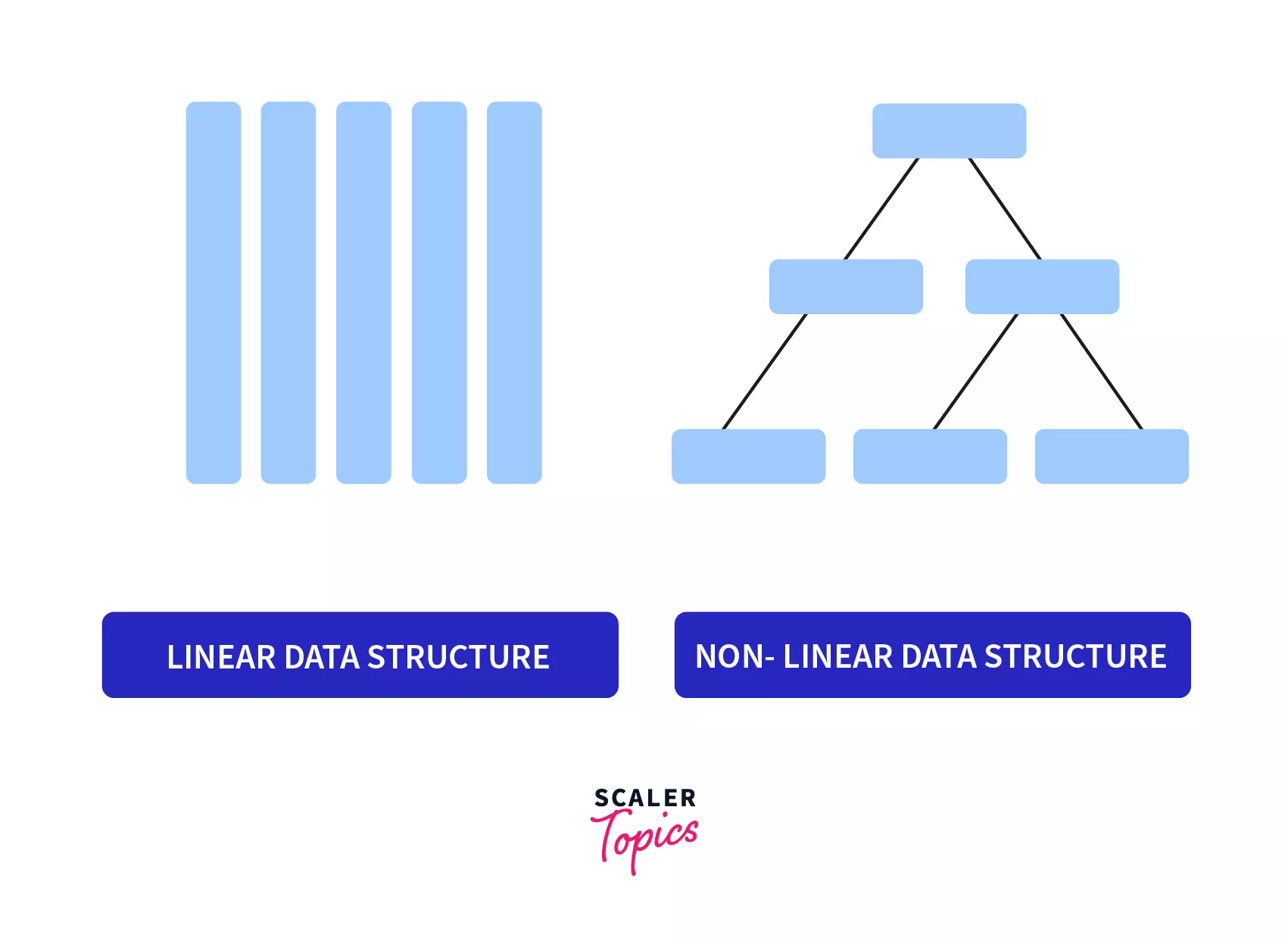Linear Data StructureNon Linear Data Structure
Elements are connected sequentially or in a contiguous manner.Elements are not connected sequentially or in a contiguous manner.
Elements are always present in a single levelElements may be present in single or multiple levels.
There is no hierarchy between the elements.There is usually a hierarchy between elements.
They are easier to implement.They have a more complex implementation.
Memory allocation is sequential.Memory allocation isn't sequential.
Can be traversed in a single run.Requires multiple runs for traversal.
Inefficient utilisation of memory.Memory is utilised efficiently.
Examples include arrays, hash tables,stack, queue etc.Examples include trees, graphs etc.

## Conclusion

• Congratulations! Now you have an intuitive idea of what non linear data structures are, and how they differ from the linear data structures out there.
• Feel free to dive deeper into the implementations of the non linear data structures you learnt about today, and take up a step up in your Data Structures journey.
Challenge Time!Time to test your skills and win rewards! Note: Rewards will be credited after the next product update.